﻿

### Some commutativity theorems through a Streb\'s classification

#### Abstract

In the present paper we investigate commutativity of rings with unity satisfying any one of the properties(Error rendering LaTeX formula),(Error rendering LaTeX formula),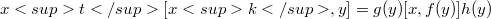$xt[xk,y]=g(y)[x,f(y)]h(y)$ and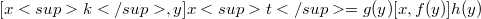$[xk,y]xt=g(y)[x,f(y)]h(y)$ for some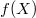$f(X)$ in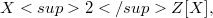$X2Z[X],$ where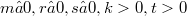$m≥ 0, r≥ 0, s≥ 0, k>0, t>0$ are non-negative integers. Finally, under different appopriate constraints on commutators, commutativity of R has been established.

DOI Code: 10.1285/i15900932v14n1p71

Full Text: PDF